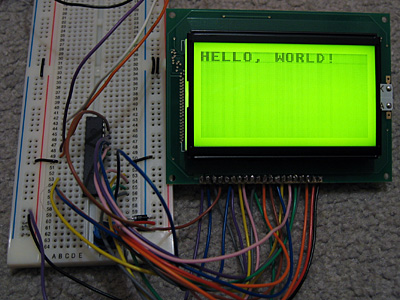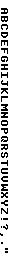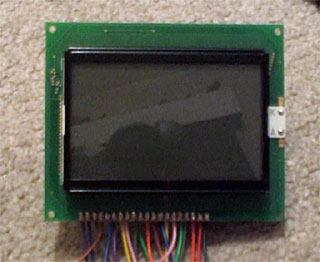Jump to content
• Advertisement• entries
222
• comments
606
• views
592238

# Back to Hardware

I enjoy dabbling with low-level programming, but have never actually built a computer to run these programs. I think it's time to correct that, and as the BBC BASIC project has required me to develop an almost complete Z80 OS (the only thing that's left for the TI-OS to do is manage files) I thought a Z80 computer would be a good start.

The planned specs are (as a starting point):
• 10 MHz Z80180 CPU;
• 64KB RAM (2 32Kx8 SRAM chips);
• 128KB Flash ROM;
• Graphical LCD;
• Simple joypad input;
• Keyboard input (AT using either software AT routines or dedicated microcontroller).
The first spanner in the works is the Z80180, as I didn't read the datasheet closely enough and it's in a DIP 64 package with 0.07" pin spacing instead of the standard 0.1" pin spacing. I'll need to find some way of constructing an adapter so I can use it with my breadboards and stripboard. [smile]

In the meantime, I've concentrated on the graphical LCD. I picked a 128x64 backlit graphical LCD for the princely sum of GBP16. It's very easy to control - you hook up it up to a 8-bit data bus to transfer image data and instructions and a handful of control pins to indicate what you're doing on that bus (reading or writing, whether you're sending an instruction or some image data, that sort of thing) and that's it - the only supporting circuitry it requires is a 10K potentiometer to act as a contrast control and power for the display and backlight.To experiment with the LCD, I'm using a PICAXE-28X1 microcontroller, programmed in BASIC. There isn't much space to store graphics, so I'm using a 32 character font (at eight bytes per character, that takes up all 256 bytes of free EEPROM space!)

; LCD data bus should be connected to port C.Symbol LcdRegisterSelection = 0 ; D/I  :  4Symbol LcdReadWrite         = 1 ; R/W  :  5Symbol LcdStartEnable       = 2 ; E    :  6Symbol LcdChipSelect1       = 3 ; CS1  : 15Symbol LcdChipSelect2       = 4 ; CS2  : 16Symbol LcdReset             = 5 ; /RST : 17; Storage for console state variables.Symbol ConsoleX             = B10Symbol ConsoleY             = B11Symbol ConsoleChar          = B12	GoSub LcdInit                              ; Initialise LCD.	B0 = %00111111 : GoSub LcdWriteInstruction ; Switch LCD on.	GoSub LcdClear ; Clear LCD		; Write the obligatory message to the LCD.		ConsoleX = 0 : ConsoleY = 0	ConsoleChar = $08 : GoSub LcdPutChar ; H ConsoleChar =$05 : GoSub LcdPutChar ; E	ConsoleChar = $0C : GoSub LcdPutChar ; L ConsoleChar =$0C : GoSub LcdPutChar ; L	ConsoleChar = $0F : GoSub LcdPutChar ; O ConsoleChar =$1D : GoSub LcdPutChar ; ,	ConsoleChar = $00 : GoSub LcdPutChar ; ConsoleChar =$17 : GoSub LcdPutChar ; W	ConsoleChar = $0F : GoSub LcdPutChar ; O ConsoleChar =$12 : GoSub LcdPutChar ; R	ConsoleChar = $0C : GoSub LcdPutChar ; L ConsoleChar =$04 : GoSub LcdPutChar ; D	ConsoleChar = $1B : GoSub LcdPutChar ; ! Pause 2000 B2 = 0 MainLoop: B2 = B2 - 1 B0 = B2 GoSub LcdGotoZ Pause 30 GoTo MainLoopLcdInit: DirsC =$00               ; Set data bus to input.	High LcdStartEnable       ; We're not writing anything.	High LcdChipSelect1	High LcdChipSelect2	Low LcdReset	Pause 500	High LcdReset	Pause 500	ReturnLcdWriteInstruction:	Low LcdReadWrite	DirsC = $FF ; Data bus = output. PinsC = B0 ; Set data bus state. Low LcdRegisterSelection ; Instruction, not data. Low LcdStartEnable High LcdStartEnable DirsC =$00               ; Leave data bus floating.	ReturnLcdWriteData:	Low LcdReadWrite	DirsC = $FF ; Data bus = output. PinsC = B0 ; Set data bus state. High LcdRegisterSelection ; Data, not instruction. Low LcdStartEnable High LcdStartEnable DirsC =$00               ; Leave data bus floating.	ReturnLcdGotoX:	B0 = B0 And 7	B0 = B0 + %10111000	GoTo LcdWriteInstruction	LcdGotoY:	B0 = B0 And 63	B0 = B0 + %01000000	GoTo LcdWriteInstructionLcdGotoZ:	B0 = B0 And 63	B0 = B0 + %11000000	GoTo LcdWriteInstructionLcdClear:	For B2 = 0 To 7		B0 = B2		GoSub LcdGotoX		B0 = 0		GoSub LcdGotoY		B0 = 0		For B3 = 0 To 63			GoSub LcdWriteData		Next	Next B2	ReturnLcdPutMap:	B1 = B0 * 8	For B2 = 0 To 7		Read B1, B0		GoSub LcdWriteData		B1 = B1 + 1	Next B2	ReturnLcdPutChar:	B0 = ConsoleY	GoSub LcdGotoX	B0 = ConsoleX * 8	If B0 < 64 Then		Low LcdChipSelect2	Else		Low LcdChipSelect1		B0 = B0 - 64	EndIf	GoSub LcdGotoY	B0 = ConsoleChar	GoSub LcdPutMap	High LcdChipSelect1	High LcdChipSelect2	ConsoleX = ConsoleX + 1	If ConsoleX = 16 Then		ConsoleX = 0		ConsoleY = ConsoleY + 1		If ConsoleY = 8 Then			ConsoleY = 0		EndIf	EndIf	Return	; FontEEPROM $00,($00,$00,$00,$00,$00,$00,$00,$00,$7E,$7F,$09,$09,$7F,$7E,$00,$00)EEPROM$10,($7F,$7F,$49,$49,$7F,$36,$00,$00,$3E,$7F,$41,$41,$63,$22,$00,$00)EEPROM $20,($7F,$7F,$41,$63,$3E,$1C,$00,$00,$7F,$7F,$49,$49,$49,$41,$00,$00)EEPROM$30,($7F,$7F,$09,$09,$09,$01,$00,$00,$3E,$7F,$41,$49,$7B,$3A,$00,$00)EEPROM $40,($7F,$7F,$08,$08,$7F,$7F,$00,$00,$41,$41,$7F,$7F,$41,$41,$00,$00)EEPROM$50,($20,$61,$41,$7F,$3F,$01,$00,$00,$7F,$7F,$1C,$36,$63,$41,$00,$00)EEPROM $60,($7F,$7F,$40,$40,$40,$40,$00,$00,$7F,$7F,$06,$1C,$06,$7F,$7F,$00)EEPROM$70,($7F,$7F,$0C,$18,$7F,$7F,$00,$00,$3E,$7F,$41,$41,$7F,$3E,$00,$00)EEPROM $80,($7F,$7F,$09,$09,$0F,$06,$00,$00,$3E,$7F,$41,$31,$6F,$5E,$00,$00)EEPROM$90,($7F,$7F,$09,$19,$7F,$66,$00,$00,$26,$6F,$49,$49,$7B,$32,$00,$00)EEPROM $A0,($01,$01,$7F,$7F,$01,$01,$00,$00,$3F,$7F,$40,$40,$7F,$3F,$00,$00)EEPROM$B0,($1F,$3F,$60,$60,$3F,$1F,$00,$00,$7F,$7F,$30,$1C,$30,$7F,$7F,$00)EEPROM $C0,($63,$77,$1C,$1C,$77,$63,$00,$00,$07,$0F,$78,$78,$0F,$07,$00,$00)EEPROM$D0,($61,$71,$59,$4D,$47,$43,$00,$00,$00,$00,$5F,$5F,$00,$00,$00,$00)EEPROM $E0,($02,$03,$59,$5D,$07,$02,$00,$00,$00,$80,$E0,$60,$00,$00,$00,$00)EEPROM$F0,($00,$00,$60,$60,$00,$00,$00,$00,$07,$07,$00,$07,$07,$00,$00,$00)

The code isn't very robust - it doesn't check the state of the LCD's busy flag as I'm assuming that a 4MHz PIC running an interpreted BASIC is too slow to manage to write another byte to the LCD driver before it has finished processing the last one.

The font was generated from the following image (it's the BBC Micro font):It's rotated through 90? as, unlike the LCD driver in the TI-83+, each byte written outputs 8 pixels vertically, with the least significant at the top. (On the TI-83+, each byte written outputs 8 pixels horizontally, with the most significant bit on the left). More interestingly, this graphical LCD is made up of two 64x64 regions next to eachother, and by controlling two chip select pins you can control whether each byte written updates the left side, the right side, neither or both. I'm entirely sure how I could use this, though, other than not-very-exciting tricks like clearing the LCD extra-fast.924KB WMV

Finally, here's a video of the LCD test in action. It's not very speedy, but will hopefully pick up some speed once I figure out how I'm going to use that Z80180 CPU. [smile]

## Recommended Comments

There are no comments to display.

## Create an account or sign in to comment

You need to be a member in order to leave a comment

## Create an account

Sign up for a new account in our community. It's easy!

Register a new account

## Sign in

Already have an account? Sign in here.

Sign In Now
• Advertisement
• Advertisement
• ### Blog Entries

• Advertisement
×

## Important Information

By using GameDev.net, you agree to our community Guidelines, Terms of Use, and Privacy Policy.

GameDev.net is your game development community. Create an account for your GameDev Portfolio and participate in the largest developer community in the games industry.

Sign me up!